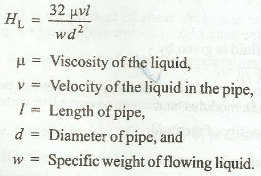# Newton's Law of Viscosity

According to Newton's law of viscosity, the shear stress on a layer of a fluid is directly proportional to the rate of shear strain. Ideal fluid is a fluid with zero viscosity. Real fluids possess viscosity.

The following important points may be noted for viscous flow:

(a) A fluid which has no viscosity is known as an ideal fluid.

(b) A fluid which has a viscosity is known as a real fluid.

(c) A fluid whose viscosity does not change with the rate of deformation or shear strain is known as Newtonian fluid.

(d) A fluid whose viscosity changes with the rate of deformation or shear strain is known as Non-Newtonian fluid.

(e) A fluid in which the viscosity of fluid is dominating over the inertia forces, is called laminar flow. It takes place at very low velocities.

(f) A flow in which the inertia force is dominating over the viscosity, is called turbulent flow. It takes place at high velocities.

(g) The velocity at which the flow changes from the laminar flow to the turbulent flow, is called critical velocity. It is of two types, i.e., lower critical velocity and higher critical velocity. The velocity at which the laminar flow stops, is known as lower critical velocity, while the velocity at which the turbulent flow starts, is known as higher Critical velocity.

(h) The ratio of the inertia force to the viscous force is called Reynold's Number. The flow in a pipe is laminar when Reynold's number is less than 2000 and flow is turbulent when Reynold's number is more than 2800. But when Reynold's number is between 2000 and 2800, the flow is neither laminar nor turbulent.

(i) The velocity corresponding to Reynold's number of 2000, is called lower critical velocity and the velocity corresponding to Reynold's number of 2800, is called higher critical velocity.

(j) The loss of head due to viscosity for laminar flow in pipe is(k) The loss of head due to friction in a pipe of uniform diameter in which a viscous flow is taking place is 16/Rn, where Rn is Reynold's number.

### You may like these posts:

1.2.